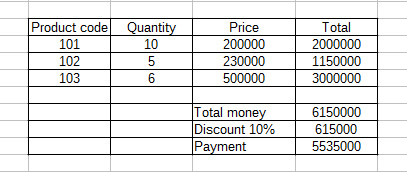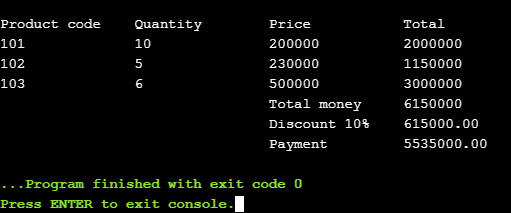# Enter and display invoice information in C plus using arrays

in Others

A simple example of information input and output in C++ using arrays, we will talk about an actual sales invoice example.

Subject: Show sales invoice information on the screen as the following picture.

– Enter invoice information including invoice code, quantity of goods, unit price.
– Calculate the total amount of each bill, discount and the amount to be paid.Show sales invoice information on the screen as picture.

Solution:

The problem contains simple information, but you need a 2-dimensional array to handle this problem. This 2-dimensional array includes:
– Number of invoices to enter.
– Number of entries to enter, specifically in this article will be 3 (Invoice code, number of rows, unit price).
– Write code for input and output information in the same function main.
– Use the for function to enter information and save it in a 2-dimensional array.
– And use the for function to display as required post.

#include

using namespace std;

int main()
{
int numRows = 0;
int numCols{ 3 };
int value = 0;
string inputStr = “”;

cout << “Number of invoices required: “;
cin >> numRows;

// Declare a [input value, 3] array
int numArray[numRows][numCols]{};

for (int row{ 0 }; row < numRows; ++row)
{
for (int col{ 0 }; col < numCols; ++col)
{
if (col == 0) {
inputStr = “Enter the product code “;
}
if (col == 1) {
inputStr = “Enter the quantity “;
}
if (col == 2) {
inputStr = “Enter the unit price “;
}

cout << inputStr << row + 1 << “: [” << row << “] [” << col << “] = “;
cin >> value;
numArray[row][col] = value;
}
}

cout << “\nProduct code\tQuantity\tPrice\t\tTotal\n” ;
int total = 0;

// Print result
for (int row{ 0 }; row < numRows; ++row)
{
for (int col{ 0 }; col < numCols; ++col)
{
cout << numArray[row][col] << “\t\t”;
}
cout << numArray[row] * numArray[row];

total = total + numArray[row] * numArray[row];

cout << ‘\n’;
}
cout << “\t\t\t\tTotal money\t” << total << “\n” ;

cout << “\t\t\t\tDiscount 10%\t”;
float discount = total * 0.1;
printf (“%.2f”, discount);
cout << “\n”;

cout << “\t\t\t\tPayment\t\t”;
float payment = total – discount;
printf(“%.2f”, payment);

return 0;
}

The result returns 0 is the success of the int main function.

Result:Suggestions:

C ++ is a fast, powerful language that is widely used in many fields and never seems to be an outdated language, you can write C ++ without having to install the environment, the editor, … by use Complier online here: https://www.onlinegdb.com/online_c++_compiler.

Hope the above example helps you on using arrays in C++.

Tags: , , , , ,